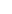Continuing with our previous example, we consider that the price of the coin falls further at 0.00005000 BTC. We recall that our sell order concerns 200 coins at 0.00005850 BTC. Let's assume that the option of buying 200 more coins at the current price now seems either that it has a high cost for our financial means, or that it involves more risk than we are willing to take. So, we can do the following:

• Depending on the money we want to continue to invest, we divide the existing sell order into equal parts. Suppose we divide our order into four orders of 50 coins each.

• We place the 3 of them at the same price as we have placed them before, that is, at 0.00005850 BTC and keep 50 coins without placing them at any sell order .

•We recall that the cost of each coin we hold is 0.00005700 BTC.

• We calculate the gain that is proportional to 50 coins: 0.00030000 / 4 = 0.00007500

• We buy 50 more at 0.00005000 BTC and the average cost is now set at (0.00005000 + 0.00005700) / 2 = 0.00005350 BTC.

• And again applying the same strategy we previously had, we place a new 100-piece sell order at (100 x 0.00005350) + 0.00007500) / 100 = 0.00005425 BTC.

What we achieve with this strategy is that we are still close to the current price, without engaging ever-increasing capital and saving resources to continue to follow it if the fall continues.to signal pageAveraging Down Strategy In Segments ❺❺❺ - average rating 5 from 5 (based on 203 user reviews)